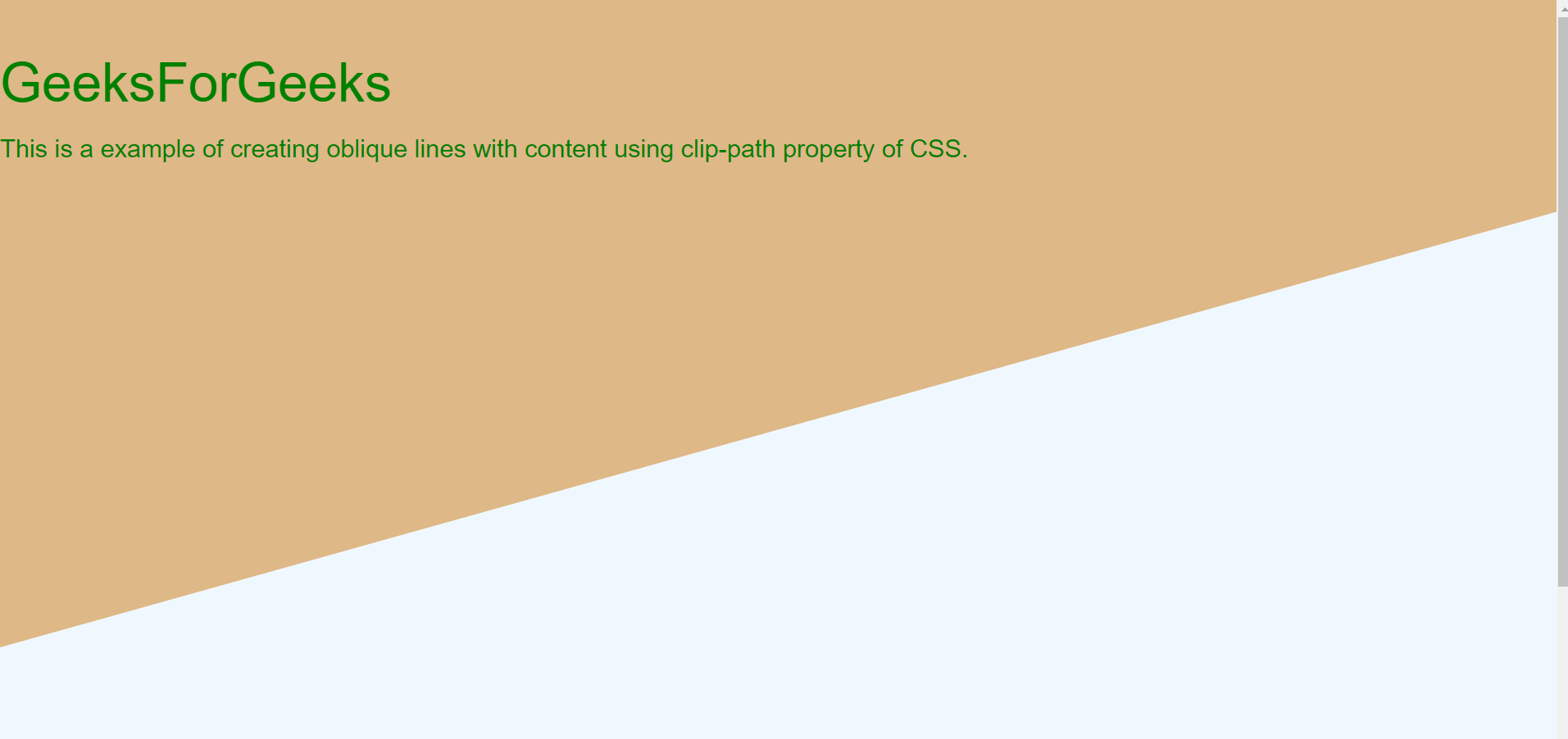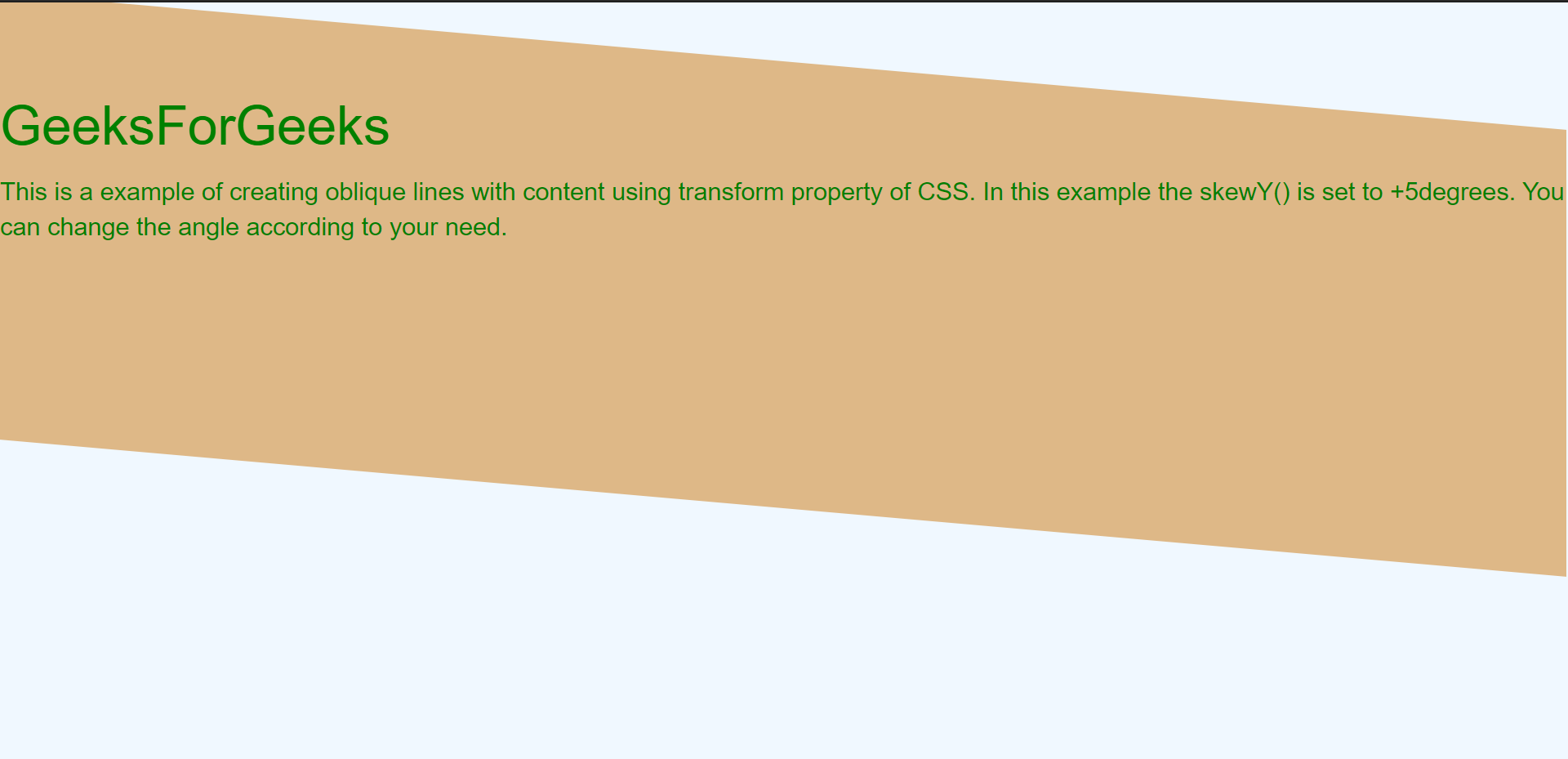Related Articles
How to Make Oblique Lines with CSS / Bootstrap 3 ?
• Last Updated : 16 Nov, 2020

In order to make oblique lines with CSS, there are two main approaches. The first approach involves the clip-path property of CSS and in the second approach, we use transform property with skew() of CSS.

Approach 1: Using clip-path property: The clip-path property is generally used to clip an element to a basic shape. But it can also be used to create oblique lines with tweaking property of polygon shape of clip-path. The main disadvantage of this property is its implementation and since many changes are needed to be observed and changed for converting it into oblique lines.

## HTML

 ` ` `<``html` `lang``=``"en"``> ` `<``head``> ` `    ``<``meta` `charset``=``"UTF-8"``> ` `    ``<``meta` `name``=``"viewport"` `content``= ` `        ``"width=device-width, initial-scale=1.0"``> ` ` `  `    ``<``title``>Oblique Lines in CSS ` ` `  `    ``<``link` `rel``=``"stylesheet"` `href``= ` `"https://maxcdn.bootstrapcdn.com/bootstrap/3.3.7/css/bootstrap.min.css"` `        ``integrity``= ` `"sha384-BVYiiSIFeK1dGmJRAkycuHAHRg32OmUcww7on3RYdg4Va+PmSTsz/K68vbdEjh4u"` `        ``crossorigin``=``"anonymous"``> ` ` `  `    ``<``style``> ` `        ``body{ ` `            ``background-color: burlywood; ` `            ``margin:0; ` `          ``} ` `          ``div.polygon{ ` `            ``height: 100vh; ` `            ``width: 100vw; ` `            ``background: aliceblue; ` `            ``clip-path: polygon(0 60%,  ` `                ``100% 3%, 100% 100%, 0 75%); ` `            ``display:flex; ` `          ``} ` `           `  `          ``.content{ ` `            ``height: 50%; ` `            ``width: 100%; ` `            ``padding-top: 10px; ` `            ``margin: auto; ` `            ``color: green; ` `          ``} ` `    `` ` ` ` ` `  `<``body``> ` `    ``<``div` `class``=``"content"``> ` `        ``<``h2``>GeeksForGeeks ` `         `  `        ``<``p``> ` `            ``This is a example of creating  ` `            ``oblique lines with content  ` `            ``using clip-path property of CSS.  ` `        `` ` `    ``   ` ` `  `    ``<``div` `class``=``"polygon"``> ` ` ` ` `  ``

Output:

The output image contains two oblique lines this is the zoomed output of the above part.Using clip-path property and polygon as shape

Approach 2: Using transform property: The transform skew property of CSS helps in rotating the division along x and y-axis. The skewX() and skewY() takes degrees of rotation as input. This property implementation is simple as compared to clip-path.  Also, if you don’t want to rotate the content then we need to rotate in the reverse direction of division.

## HTML

 ` ` `<``html` `lang``=``"en"``> ` ` `  `<``head``> ` `    ``<``meta` `charset``=``"UTF-8"``> ` `    ``<``meta` `name``=``"viewport"` `content``= ` `        ``"width=device-width, initial-scale=1.0"``> ` ` `  `    ``<``title``>Oblique line using CSS ` ` `  `    ``<``link` `rel``=``"stylesheet"` `href``= ` `"https://maxcdn.bootstrapcdn.com/bootstrap/3.3.7/css/bootstrap.min.css"` `        ``integrity``= ` `"sha384-BVYiiSIFeK1dGmJRAkycuHAHRg32OmUcww7on3RYdg4Va+PmSTsz/K68vbdEjh4u"` `        ``crossorigin``=``"anonymous"``> ` ` `  `    ``<``style``> ` `        ``body { ` `            ``background-color: aliceblue; ` `        ``} ` ` `  `        ``.maintransform { ` `            ``margin-right: 1px; ` `            ``margin-left: 0px; ` `            ``background-color: burlywood; ` `            ``position: absolute; ` `            ``top: 33px; ` `            ``left: 0px; ` `            ``right: 0px; ` `            ``height: 250px; ` `            ``color: green; ` `            ``font-family: Arial, Helvetica, sans-serif; ` `            ``-ms-transform: skewY(5deg); ` `            ``-webkit-transform: skewY(5deg); ` `            ``transform: skewY(5deg); ` `        ``} ` ` `  `        ``.content { ` `            ``-ms-transform: skewY(5deg); ` `            ``-webkit-transform: skewY(5deg); ` `            ``transform: skewY(-5deg); ` `            ``padding-left: 0px; ` `        ``} ` `    `` ` ` ` ` `  `<``body``> ` ` `  `    ``<``div` `class``=``"maintransform"``> ` `        ``<``h2` `class``=``"content"``>GeeksForGeeks ` ` `  `        ``<``p` `class``=``"content"``> ` `            ``This is a example of creating oblique  ` `            ``lines with content using transform  ` `            ``property of CSS. In this example  ` `            ``the skewY() is set to +5degrees.  ` `            ``You can change the angle according  ` `            ``to your need. ` `        `` ` `    `` ` ` ` ` `  ``

Output:

Here the rotation is around the y-axis and skew angle is +5(deg) whereas the text angle is at -5(deg) to make the text straight.My Personal Notes arrow_drop_up
Recommended Articles
Page :